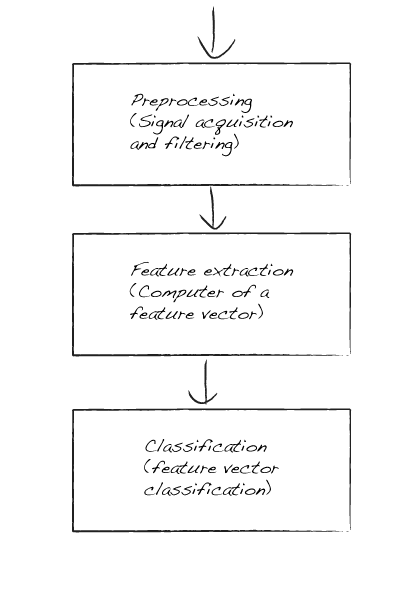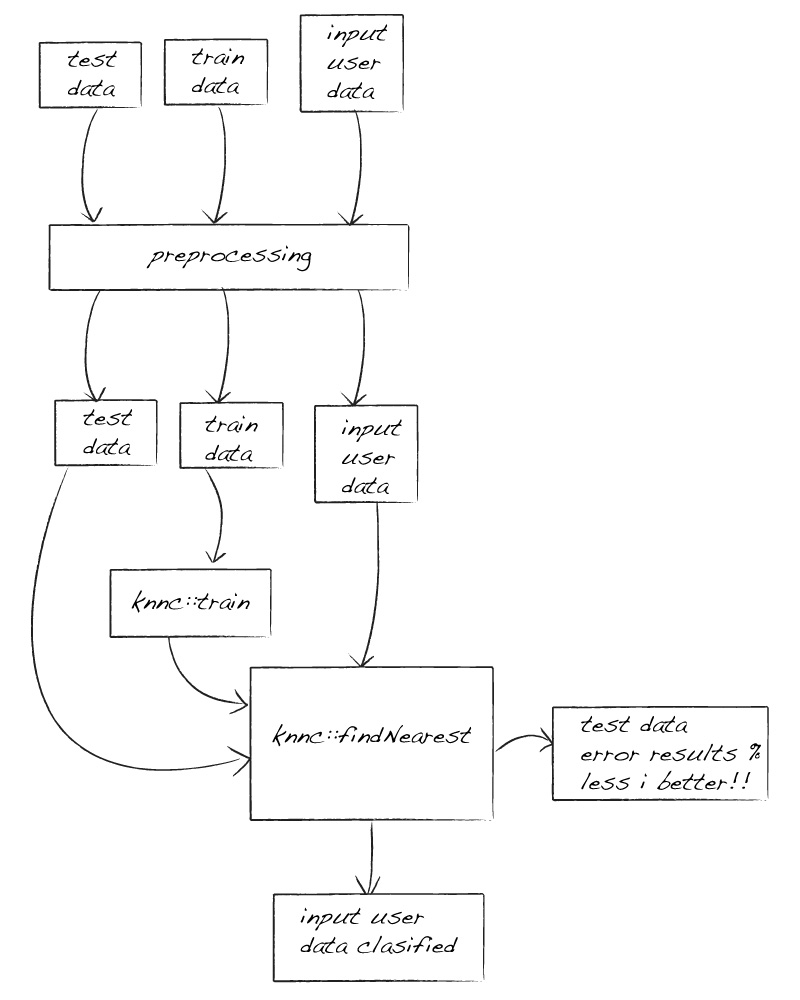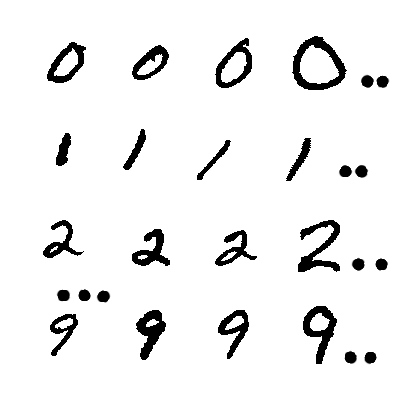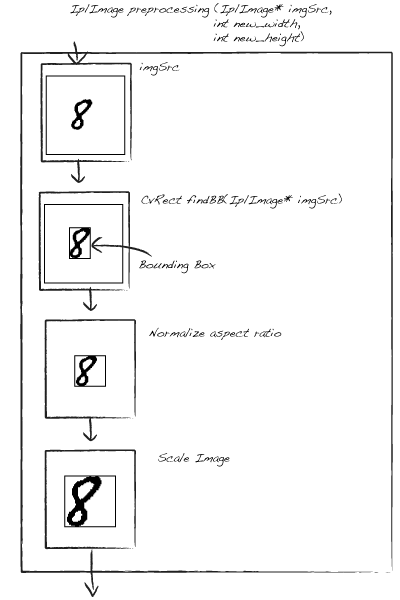In this tutorial we go to create a basic number OCR. It consist to classify a handwrite number into his class. To do it, we go to use all we learn in before tutorials, we go to use a simple basic painter and the basic pattern recognition and classification with openCV tutorial.

Demo Source from GitHub

In a typical pattern recognition classifier consist in three modules:Preprocessing: in this module we go to process our input image, for example size normalize, convert color to BN…

Feature extraction: in this module we convert our image processed to a characteristic vector of features to classify, it can be the pixels matrix convert to vector or get contour chain codes data representation

Classification module get the feature vectors and train our system or classify an input feature vector with a classify method as knn.

In this basic OCR we go to use this graph:Where we get a train set and test set of image to train and test our classifier method (knn)

We have a 1000 handwrite images, 100 images of each number. We get 50 images of each number (class) to train and other 50 to test our system.Then the first work we do is pre-process all train image, to do it we create a preprocessing function. In this function we get a image and a new width and height we want as result of preprocessing, then the function return a normalized size with bounding box image. You can see more clear the process in this graph:Pre-processing code:

We use the function getData of basicOCR class to create the train data and train classes, this function get all images under OCR folder to create this train data, the OCR forlder is structured with 1 folder to each class and each file have are pbm files with this name cnn.pbm where c is the class {0..9} and nn is the number of image {00..99}

Each image we get is pre-processed and then convert the data in a feature vector we use.

basicOCR.cpp getData code:

After processed and get train data and classes whe then train our model with this data, in our sample we use knn method then:

Then we now can test our model, and we can use the test result to compare to another methods we can use, or if we reduce the image scale or similar. There are a function to create the test in our basicOCR class, test function.

This function get the other 500 samples and classify this in our selected method and check the obtained result.

Test use the classify function that get image to classify, process image, get feature vector and classify it with a find_nearest of knn class. This function we use to classify the input user images:

All work or training and test is in basicOCR class, when we create a basicOCR instance then only we need call to classify function to classify our input image. Then we go to use basic Painter we create before in other tutorial to user interactivity to draw a image and classify it.

Demo Source